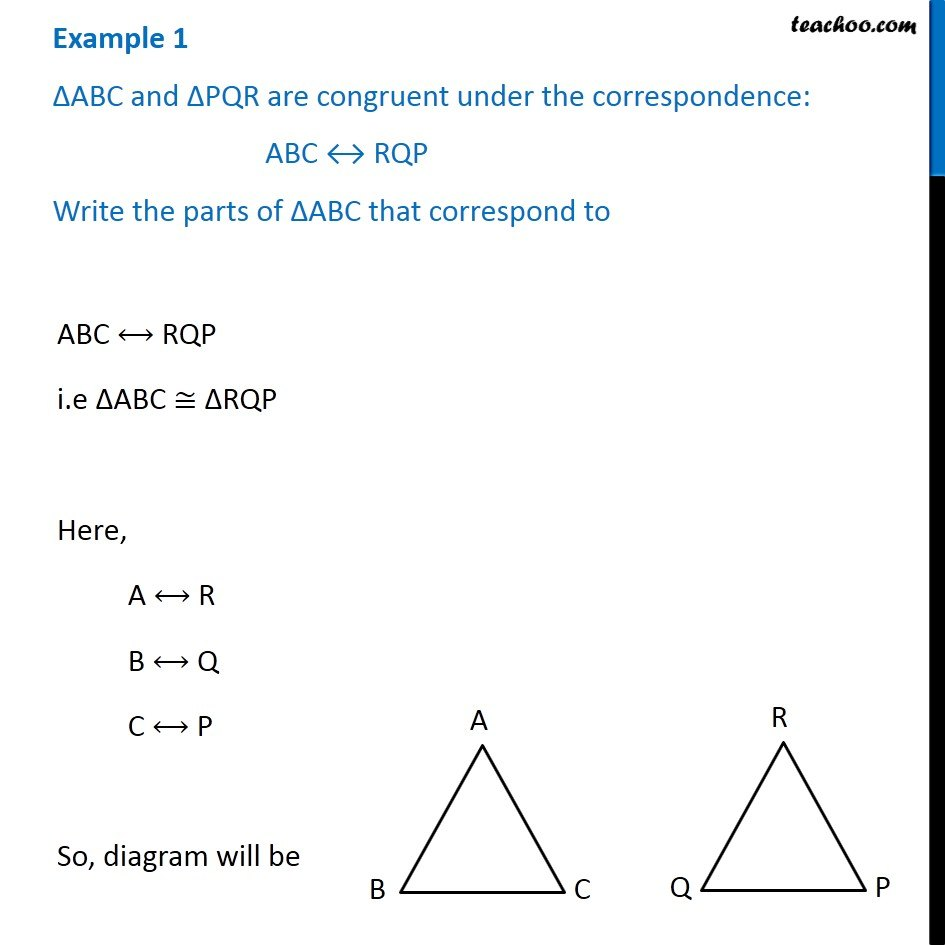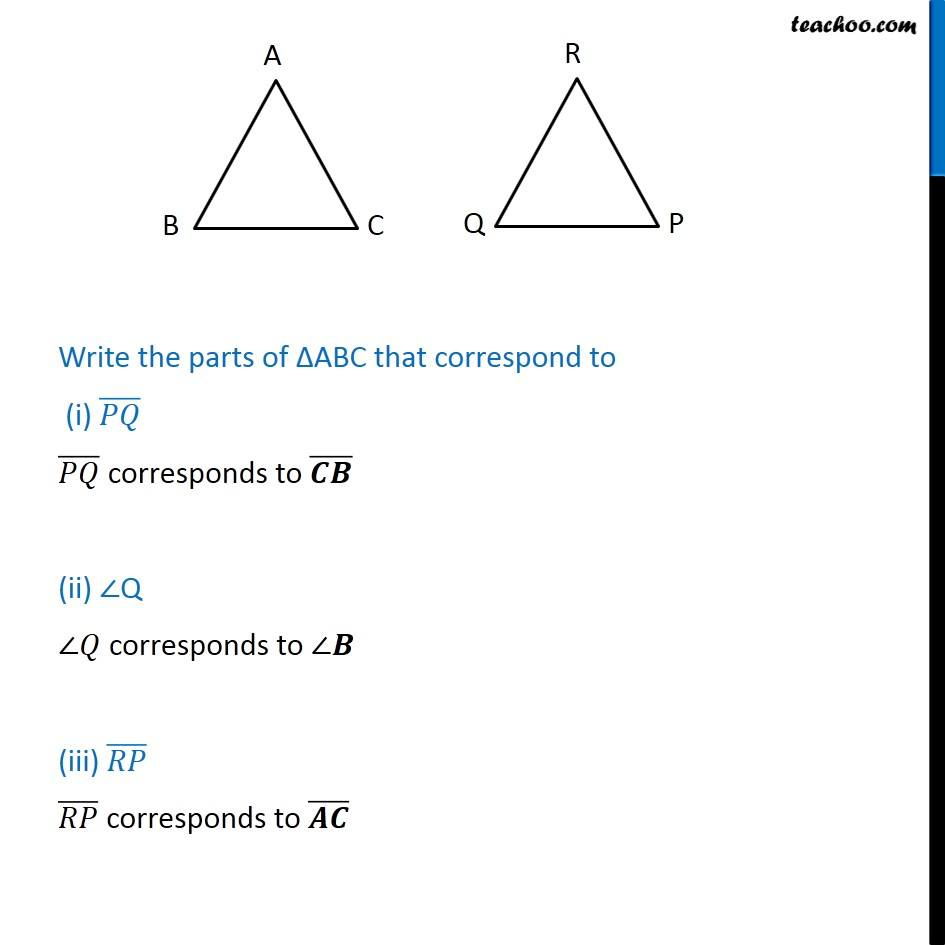Examples

Congruence of Triangles
Serial order wiseLearn in your speed, with individual attention - Teachoo Maths 1-on-1 Class

### Transcript

Example 1 - Chapter 7 Class 7 Congruence of Triangles - NCERT ∆ABC and ∆PQR are congruent under the correspondence: ABC ↔ RQP Write the parts of ∆ABC that correspond to ABC ⟷ RQP i.e ∆ABC ≅ ∆RQP Here, A ⟷ R B ⟷ Q C ⟷ P So, diagram will be Write the parts of ∆ABC that correspond to (i) (𝑃𝑄) ̅ (𝑃𝑄) ̅ corresponds to (𝑪𝑩) ̅ (ii) ∠Q ∠𝑄 corresponds to ∠𝑩 (iii) (𝑅𝑃) ̅ (𝑅𝑃) ̅ corresponds to (𝑨𝑪) ̅